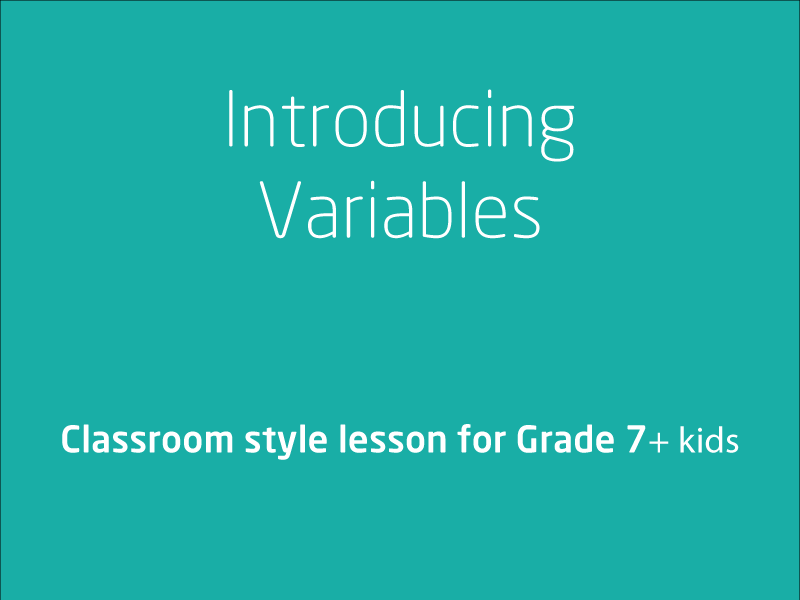Free video lessons for Foundation to Grade 8 studentsEquivalence fractions

In this video lesson, your coach will teach you how to compare fractions using equivalence. Locate and represent positive and negative fractions and mixed numbers on a number ...

1 videos
Intermediate difficulty
0 0 0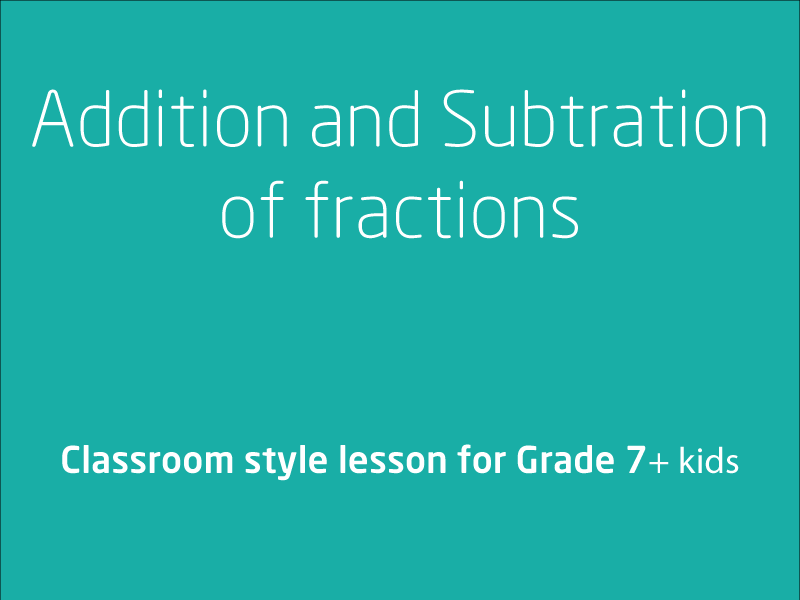In this video lesson we will be looking at solving problems involving addition and subtraction of fractions, including those with unrelated denominators. We will also be explo...

2 videos
Intermediate difficulty
0 0 0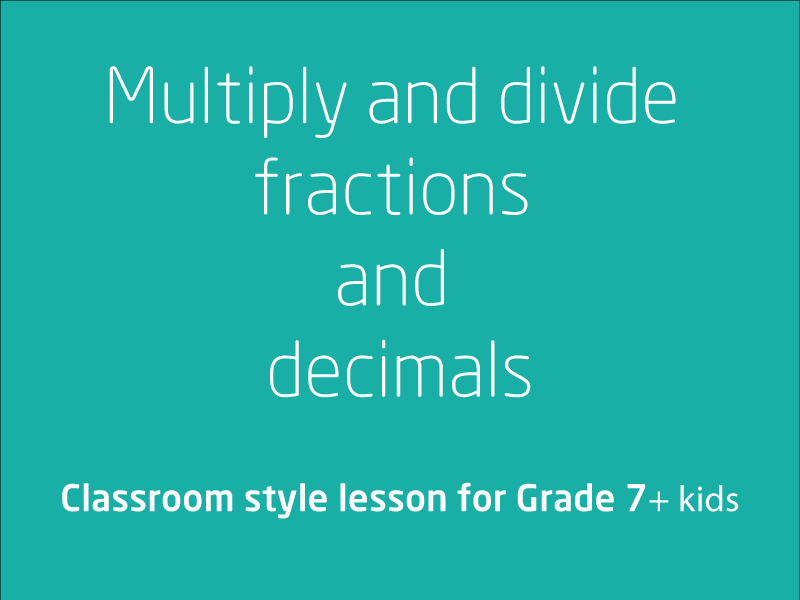Multiply and divide fractions and decimals

In this video lesson, we will be looking at techniques that will help you take your multiplication and division skills involving fractions to a new level. This is a 3 part course. ...

3 videos
Intermediate difficulty
0 0 0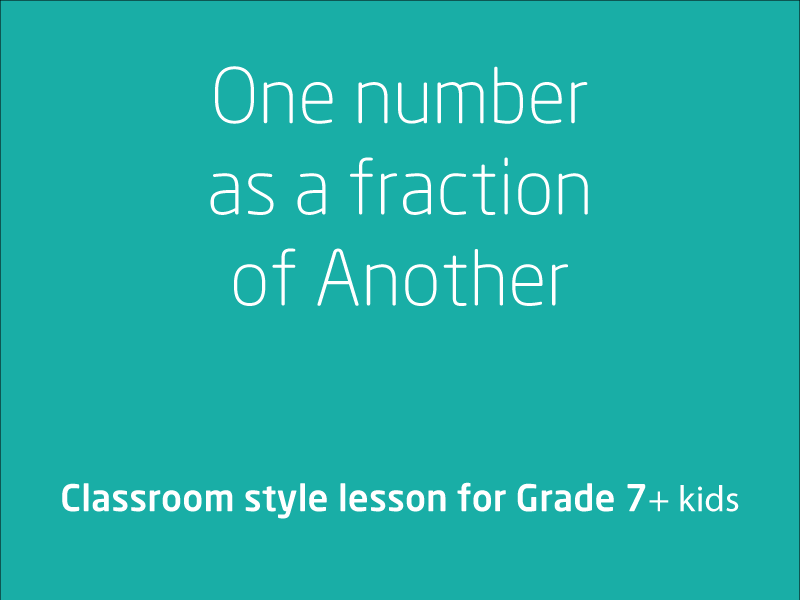Expressing one number as a fraction of another

In this video lesson we will be working through Expressing one number as a fraction of another. We will be using authentic examples for the quantities to be expressed and...

1 videos
Intermediate difficulty
0 0 0Rounding decimals to a specified number of decimal places

In this video lesson we will be exploring rounding decimals to a specific number of decimal places, using rounding to estimate the results of calculations with whole numbers a...

1 videos
Intermediate difficulty
0 0 0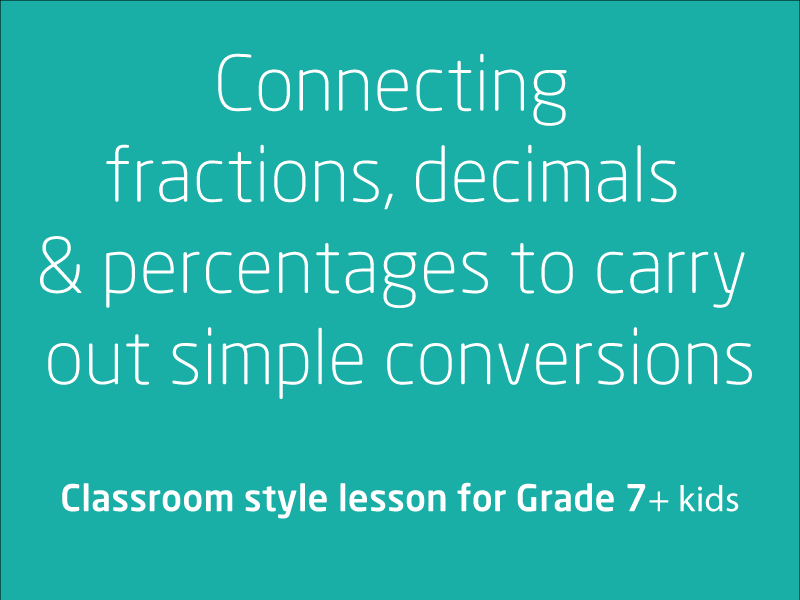Connecting fractions, decimals and percentages to carry out simple conversions

In the video lesson we will be looking at Connecting fractions, decimals and percentages to carry out simple conversions. This lesson has several examples to help you understa...

2 videos
Intermediate difficulty
0 0 0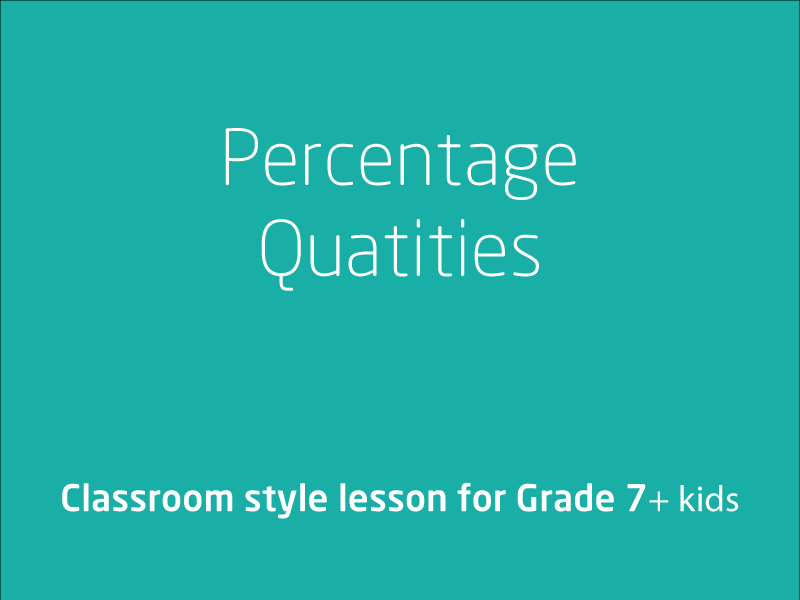Percentage quantities

In this video lesson we will focus on finding  percentages of quantities and express one quantity as a percentage of another. We recommend that student should take a few ...

2 videos
Intermediate difficulty
0 0 0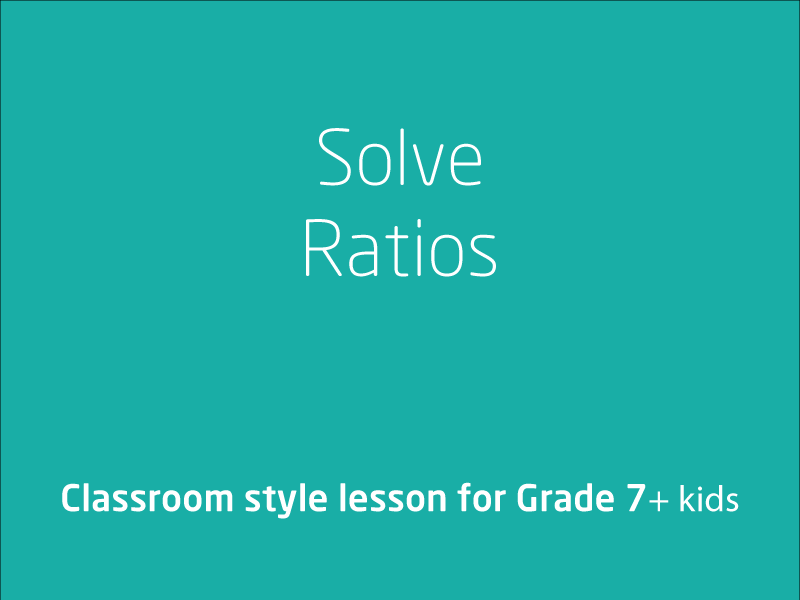Recognise and solve problems involving simple ratios

The main aim of this video lesson is to firm your understanding that rate and ratio problems can be solved using fractions or percentages and we'll be choosing the most ef...

1 videos
Intermediate difficulty
0 0 0In this video lesson we will Investigate and calculate 'best buys'Elaborationsapplying the unitary method to identify ‘best buys’ situations, such as compa...

1 videos
Intermediate difficulty